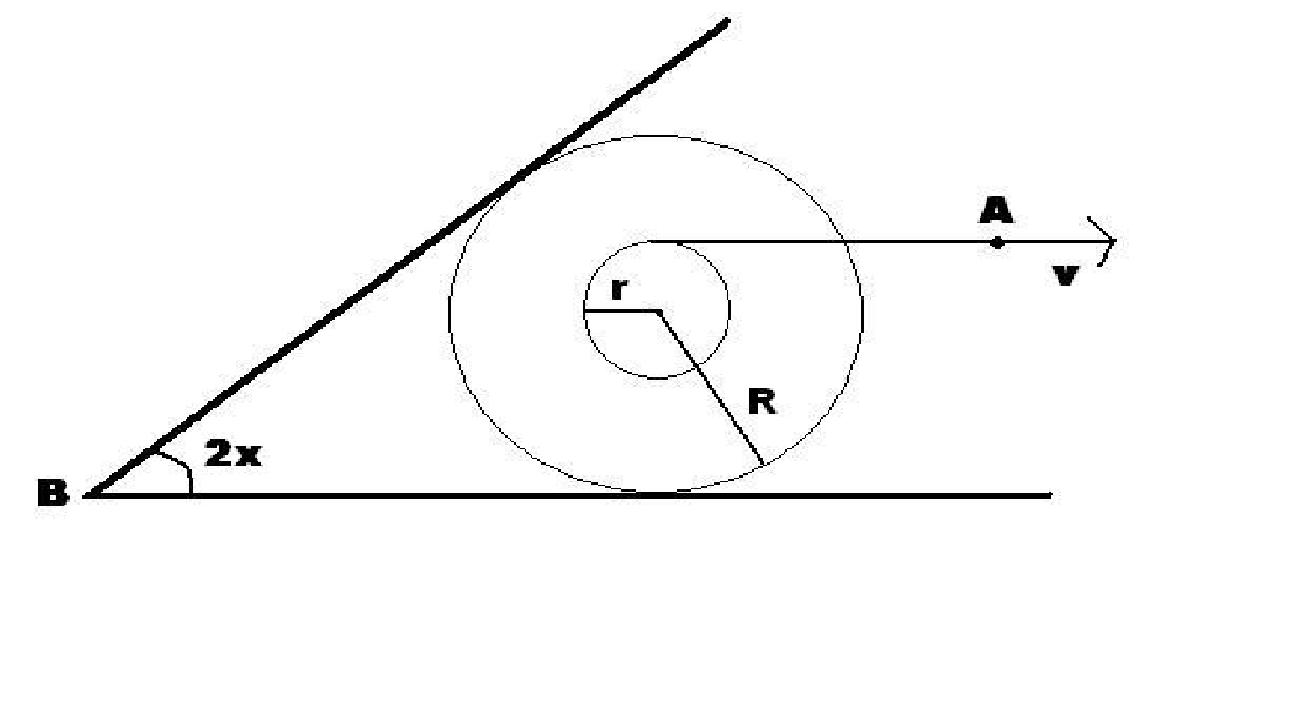# Hello Bobbin

A bobbin rolls without slipping on a horizontal surface so that the velocity $v$ of the end of the thread (point $A$) is directed along the horizontal. A board hinged at point $B$ leans against the bobbin making an angle $2x$ with the horizontal. The inner and outer radii of the bobbin are $r$ and $R$ respectively.If the angular velocity $w$ of the board can be expressed in terms of $x$ as $\dfrac{kv(\sin x)^{2}}{r+R}$, find the magnitude of $k$.

×

Problem Loading...

Note Loading...

Set Loading...